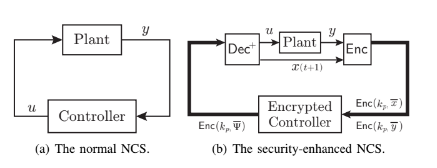# Encrypted control system using RSA

I am tying to implement an encrypted control system with Matlab using RSA encryption. The problem is that it is not working and i don’t get the correct output. In particular the scheme i am following is. My code is

``````A_p = [9.9998*(10)^-1 1.9700*(10)^-2; -1.970*(10)^-2 9.7025*(10)^-1];
``````

B_p = [9.9900*(10)^-5; 9.8508*(10)^-3]; C_p = [1 0]; D_p = 0;

X0 = [1;0]; %initial conditions for the plant

t = [0:0.01:5]; %sampling time

u = ones(1,length(t)); %encrypted input y_p = zeros(1, length(t)); %output of the plant x_p = zeros(2, length(t)); %state of the plant x_c = zeros(2, length(t)); %state of the controller

x_p(:, 1) = X0; %assign the initial values to the satet of the %plant

%parameters of the controller

A_c = [1 0.0063; 0 0.3678]; B_c = [0; 0.0063]; C_c = [10 -99.90]; D_c = 3;

end_t = length(t);

N = 94399927; % N=p*q e = 11; %public key d = 85800451; %private key

%encrypted parameters for the controller

A_enc = mod(A_c.^e, N); B_enc = mod(B_c.^e, N); C_enc = mod(C_c.^e, N); D_enc = mod(D_c.^e, N);

xc_enc = zeros(2,length(t)); %x_c encrypted y_enc = zeros(1,length(t)); %y_c encrypted

u_dec = ones(1, length(t)); %u decripted

y_quant = zeros(1, length(t));

for ind=1:end_t-1

``````%plant

x_p(:, ind+1) = A_p*x_p(: ,ind) + B_p*u_dec(ind);
y_p(ind) = C_p*x_p(: ,ind);

y_quant(ind) = uencode(y_p(ind),3); %quantization

%encryption of y_p

y_enc(ind) = modpow(y_quant(ind), e, N);;

%controller with encrypted parameter
xc_enc(:, ind+1) = A_enc*xc_enc(:, ind) - B_enc*y_enc(ind);
u(ind+1) = C_enc*xc_enc(:, ind) - D_enc*y_enc(ind);

%decrypting u
u_dec(ind+1) = modpow(u(ind+1),d,N);
``````

%function to do the modular exponentiation with big numbers in Matlab

``````function result = modpow(base,exp,m)
result = 1;
while (exp > 0)
if bitand(exp,1) > 0
result = mod((result * base),m);
end
exp = bitshift(exp,-1);
base = mod(base^2,m);
end
end
``````

end

figure plot(t,y_enc);

figure plot(t,u);

I hope it is understandable, if not please tell me i will edit. Can somebody help me? Thank’s in advance.

p.s. I am trying to implement what is written in the paper “Cyber-Security Enhancement of Networked Control Systems Using Homomorphic Encryption” of Kiminao Kogiso and Takahiro Fujita.